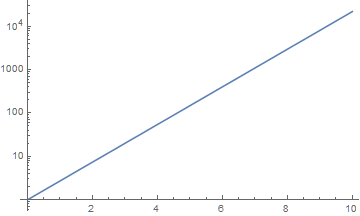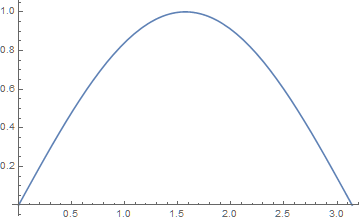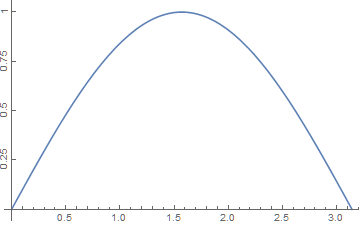# How do I manually edit tick marks on plots?

The Ticks option allows you to manually specify the ticks and labels that occur on the axes in your plots. It works for most kinds of plots in the Wolfram Language. Here are a few examples on how you can use this option to edit your tick marks.

### Creating Exponential Ticks on a LogPlot

The Wolfram Language automatically adds and positions tick mark labels on plots. Consider this LogPlot:

`LogPlot[E^x, {x, 0, 10}]`You might want to label vertical tick marks using exponents. First, create a list of the tick positions and labels that you would like to use at those positions.

`tickSpecification = Table[{10^i, Superscript[10, i]}, {i, 1, 4}]`

This produces the list of pairs:

`{{10, Superscript[10,1]}, {100, Superscript[10,2]}, {1000, Superscript[10,3]}, {10000, Superscript[10,4]}}`

Give this tick specification to the Ticks option in the LogPlot.

`LogPlot[E^x, {x, 0, 10}, Ticks -> {Automatic, tickSpecification}]`Ticks takes a list with two arguments. The first element in this example is ` Automatic`. It instructs the Wolfram Language to select the horizontal tick marks automatically.

The second element in the list is the specification for the vertical tick marks. The code above produces this LogPlot with exponential ticks.

### Rotated Ticks

For the plot below, you may want to label the vertical tick marks with rotated numbers.

`Plot[Sin[x], {x, 0, Pi}]`Doing this is similar to the first example where exponential tick marks were created. First, make a list of pairs of numbers and labels using the Table command. You can pair each number with a label of itself rotated by Pi/2 radians.

`tickSpecification = Table[{i, Rotate[i, Pi/2]}, {i, {0.25, 0.5, 0.75, 1}}]`

The Table command produces a list of pairs of numbers, where the last element of each pair is rotated. Apply the new tick specification to the plot.

`Plot[Sin[x], {x, 0, Pi}, Ticks -> {Automatic, tickSpecification}]`Notice that the positions of the tick marks are different from the default. In order to rotate the tick marks, you must first select which ticks you want, and then rotate them.

## Contact SupportCall us

## Customer Support

Monday–Friday
8am–5pm US Central Time

• Product registration or activation
• Pre-sales information and ordering
• Help with installation and first launch

## Advanced Technical Support (for eligible customers)

Monday–Thursday
8am–7pm US Central Time

Friday
8:30–10am & 11am–5pm US Central Time

• Priority technical support
• Product assistance from Wolfram experts
• Help with Wolfram Language programming